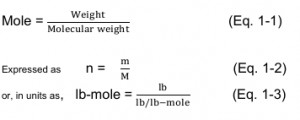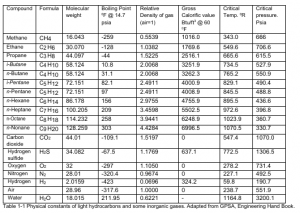# Molecular Weight and Apparent Molecular Weight

The molecular weight of a compound is the sum of the atomic weight of the various atoms making up that
compound. The Mole is the unit of measurements for the amount of substance, the number of moles is
defined as follows:Example 1.1: Methane molecule consists of one carbon atom with atomic weight =12 and 4 hydrogen atoms with atomic weight = 1 each. Molecular weight for Methane (CH 4) = (1 × 12) + (4 × 1) = 16 lb/lb-mole. Similarly, Ethane (C2H6) molecular weight = (2 × 12) + (6 × 1) = 30 lb/lb-mole.

Hydrocarbon up to four carbon atoms are gases at room temperature and atmospheric pressure. Reducing the gas temperature and/or increasing the pressure will condense the hydrocarbon gas to a liquid phase. By the increase of carbon atoms in hydrocarbon molecules, consequently the increase in molecular weight, the boiling point increases and a solid hydrocarbon is found at high molecular weight.

Physical constants of light hydrocarbon and some inorganic gases are listed in Table 1-1.